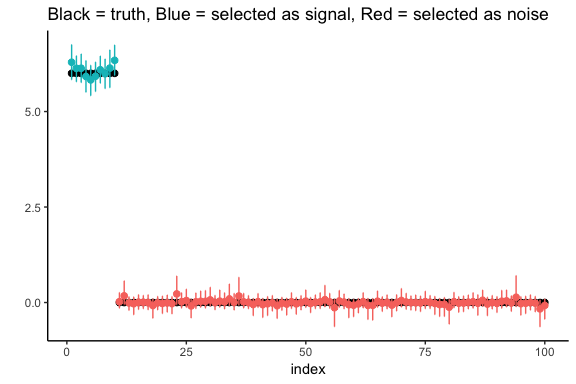Horseshoe vignette

July 18th, 2019

In this vignette, the main functions of the package horseshoe are explained. This vignette is split up into three sections:

1. Brief introduction to the horseshoe
2. The normal means problem
3. Linear regression

First, install the package by typing

install.packages("horseshoe")

and then load the package. In this vignette we also use ggplot2 to make plots.

library(horseshoe)

1. Brief introduction to the horseshoe

The horseshoe (Carvalho et al 2010) is a Bayesian method for ‘needle-in-a-haystack’ type problems where there is some sparsity, meaning that there are some signals amid mostly noise.

We introduce the horseshoe in the context of the normal means model, which is given by $Y_i = \beta_i + \varepsilon_i, \quad i = 1, \ldots, n,$ with $$\varepsilon_i$$ i.i.d. $$\mathcal{N}(0, \sigma^2)$$. The horseshoe prior is given by \begin{align*} \beta_i &\sim \mathcal{N}(0, \sigma^2 \tau^2 \lambda_i^2)\\ \lambda_i &\sim C^+(0, 1), \end{align*} where $$C^+$$ denotes the half-Cauchy distribution. Optionally, hyperpriors on $$\tau$$ and $$\sigma$$ may be specified, as is described further in the next two sections.

To illustrate the shrinkage behaviour of the horseshoe, let’s plot the posterior mean for $$\beta_i$$ as a function of $$y_i$$ for three different values of $$\tau$$.

tau.values <- c(0.005, 0.05, 0.5)
y.values <- seq(-5, 5, length = 100)
df <- data.frame(tau = rep(tau.values, each = length(y.values)),
y = rep(y.values, 3),
post.mean = c(HS.post.mean(y.values, tau = tau.values, Sigma2=1),
HS.post.mean(y.values, tau = tau.values, Sigma2=1),
HS.post.mean(y.values, tau = tau.values, Sigma2=1)) )

ggplot(data = df, aes(x = y, y = post.mean, group = tau, color = factor(tau))) +
geom_line(size = 1.5) +
scale_color_brewer(palette="Dark2") +
geom_abline(lty = 2) + geom_hline(yintercept = 0, colour = "grey") +
theme_classic() + ylab("") + labs(color = "Tau") +
ggtitle("Horseshoe posterior mean for three values of tau")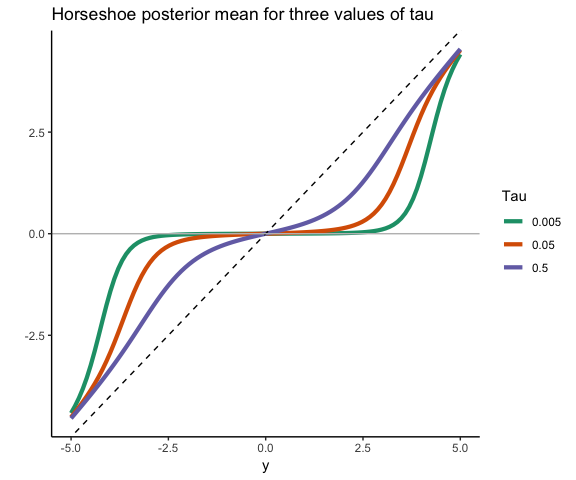Smaller values of $$\tau$$ lead to stronger shrinkage behaviour of the horseshoe. Observations that are in absolute value at most equal to $$\sqrt{2\sigma^2\log(1/\tau)}$$ are shrunk to values close to zero (Van der Pas et al (2014)). For larger observed values, the horseshoe posterior mean will tend to the identity (that is, barely any shrinkage, the estimate will be very close to the observed value). The optimal value of $$\tau$$ is the proportion of true signals. This value is typically not known in practice but can be estimated, as described further in the next sections.

2. The normal means problem

The normal means model is: $Y_i = \beta_i + \varepsilon_i, \quad i = 1, \ldots, n,$ with $$\varepsilon_i$$ i.i.d. $$\mathcal{N}(0, \sigma^2)$$.

Computing the posterior mean only, with known variance $$\sigma^2$$

The function HS.post.mean computes the posterior mean of $$(\beta_1, \ldots, \beta_n)$$. It does not require MCMC and is suitable when only an estimate of the vector $$(\beta_1, \ldots, \beta_n)$$ is desired. In case uncertainty quantification or variable selection is also of interest, or no good value for $$\sigma^2$$ is available, please see below for the function HS.normal.means.

The function HS.post.mean requires the observed outcomes, a value for $$\tau$$ and a value for $$\sigma$$. Ideally, $$\tau$$ should be equal to the proportion of nonzero $$\beta_i$$’s. Typically, this proportion is unknown, in which case it is recommended to use the function HS.MMLE to find the marginal maximum likelihood estimator for $$\tau$$.

As an example, we generate 50 data points, the first 10 of which are coming from true signals. The first 10 $$\beta_i$$’s are equal to five and the remaining $$\beta_i$$’s are equal to zero. Let’s first plot the true parameters (black) and observations (blue).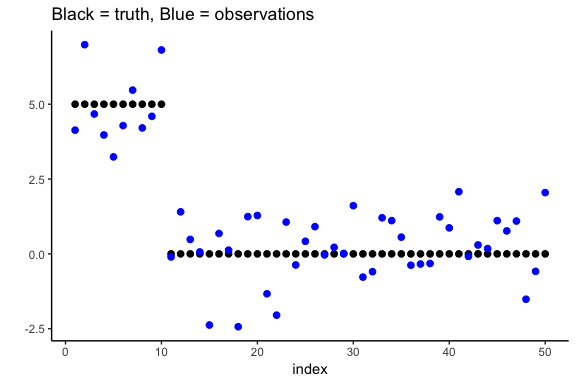We estimate $$\tau$$ using the MMLE, using the known variance.

We then use this estimate of $$\tau$$ to find the posterior mean, and add it to the plot in red.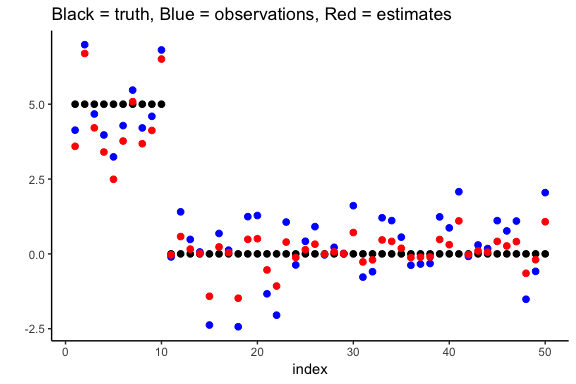If the posterior variance is of interest, the function HS.post.var can be used. It takes the same arguments as HS.post.mean.

Posterior mean, credible intervals and variable selection, possibly unknown $$\sigma^2$$

The function HS.normal.means is the main function to use for the normal means problem. It uses MCMC and results in an object that contains all MCMC samples as well as the posterior mean for all parameters ($$\beta_i$$’s, $$\tau$$, $$\sigma$$), the posterior median for the $$\beta_i$$’s, and credible intervals for the $$\beta_i$$’s.

The key choices to make are:

• How to handle $$\tau$$. The recommended option is “truncatedCauchy” (a half-Cauchy prior truncated to $$[1/n, 1]$$). See the manual for other options.
• How to handle $$\sigma$$. The recommended option is “Jeffreys” (Jeffrey’s prior). See the manual for other options.

Other options that can be set by the user are the level of the credible intervals (default is 95%), and the number of MCMC samples (default is 1000 burn-in samples and then 5000 more).

Let’s continue the example from the previous section. We first create a ‘horseshoe object’.

We extract the posterior mean of the $$\beta_i$$’s and plot them in red.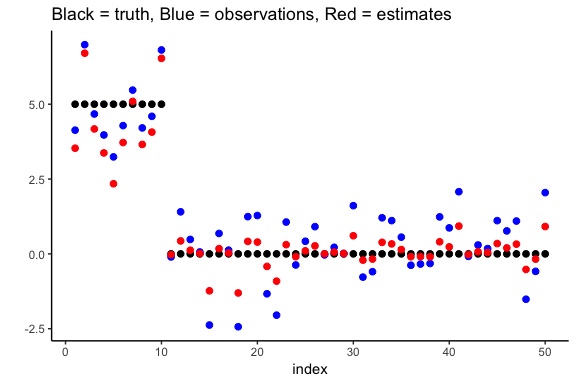We plot the marginal credible intervals (and remove the observations from the plot for clarity).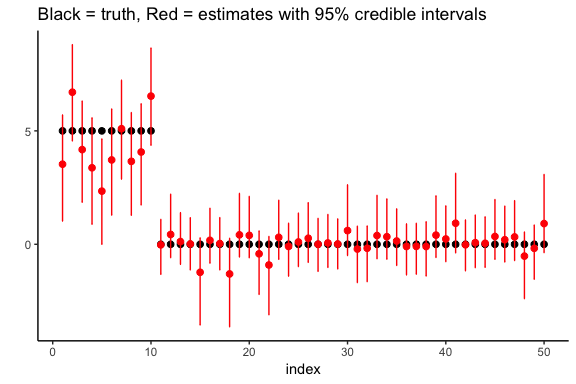Finally, we perform variable selection using HS.var.select. In the normal means problem, we can use two decision rules. We will illustrate them both. The first method checks whether zero is contained in the credible interval, as studied by Van der Pas et al (2017).

The result is a vector of zeroes and ones, with the ones indicating that the observations is suspected to correspond to an actual signal. We now plot the results, coloring the estimates/intervals blue if a signal is detected and red otherwise.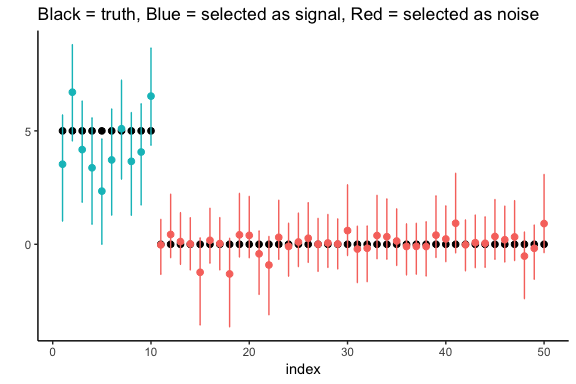The other variable selection method is the thresholding method of Carvalho et al (2010). The posterior mean can be written as $$c_iy_i$$ where $$y_i$$ is the observation and $$c_i$$ some number between 0 and 1. A variable is selected if $$c_i \geq c$$ for some user-selected threshold $$c$$ (default is $$c = 0.5$$). In the example: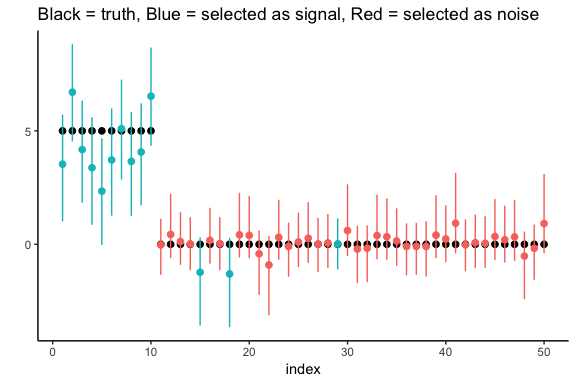3. Linear regression

The linear regression model is given by $Y = X\beta + \varepsilon,$

where $$Y$$ and $$\varepsilon$$ are vectors of length $$n$$, $$\beta$$ is a vector of length $$p$$ and $$X$$ is an $$n \times p$$-matrix. We assume $$\varepsilon \sim \mathcal{N}(0, I_n)$$. The main function for the horseshoe for the linear regression model is horseshoe and it implements the algorithm of Bhattacharya et al (2016).

The options of horseshoe are the same as for HS.normal.means (discussed above, although in case of linear regression it is less clear which prior to use for $$\tau$$). We illustrate the use of horseshoe via an example.

We create a 50 by 100 design matrix $$X$$ filled with realizations of independent normal random variables. The first 10 entries of the vector $$\beta$$ are set equal to six (the signals) and the remaining 90 entries are set equal to zero (the noise).

X <- matrix(rnorm(50*100), 50)
beta <- c(rep(6, 10), rep(0, 90))
y <- X %*% beta + rnorm(50)

We use the horseshoe and plot the posterior mean and marginal 95% credible interval per parameter in red. The true parameter values are shown in black.

hs.object <- horseshoe(y, X, method.tau = "truncatedCauchy", method.sigma ="Jeffreys")
#>  1000
#>  2000
#>  3000
#>  4000
#>  5000
#>  6000

df <- data.frame(index = 1:100,
truth = beta,
post.mean = hs.object$BetaHat, lower.CI <- hs.object$LeftCI,
upper.CI <- hs.object$RightCI ) ggplot(data = df, aes(x = index, y = truth)) + geom_point(size = 2) + theme_classic() + ylab("") + geom_point(aes(x = index, y = post.mean), size = 2, col = "red") + geom_errorbar(aes(ymin = lower.CI, ymax = upper.CI), width = .1, col = "red") + ggtitle("Black = truth, Red = estimates with 95% credible intervals")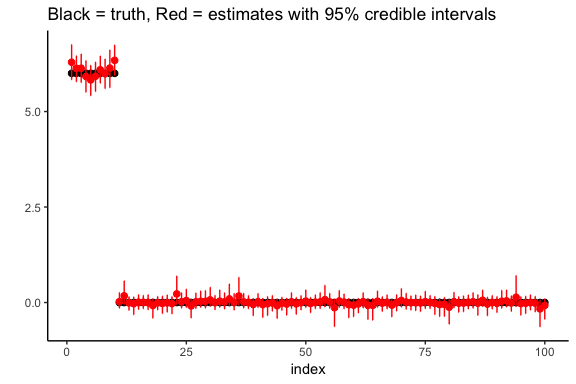We again perform variable selection. The function, HS.var.select, is the same as described above for the normal means problem. Here we show how it works when variables are selected by checking whether 0 is in the credible interval. For the thresholding procedure, please refer to the normal means example above. We perform variable selection: df$selected.CI <- HS.var.select(hs.object, df\$y, method = "intervals")

The result is a vector of zeroes and ones, with the ones indicating that the observations is suspected to correspond to an actual signal. We now plot the results, coloring the estimates/intervals blue if a signal is detected and red otherwise.

ggplot(data = df, aes(x = index, y = truth)) +
geom_point(size = 2) +
theme_classic() + ylab("") +
geom_point(aes(x = index, y = post.mean, col = factor(selected.CI)),
size = 2) +
geom_errorbar(aes(ymin = lower.CI, ymax = upper.CI, col = factor(selected.CI)),
width = .1) +
theme(legend.position="none") +
ggtitle("Black = truth, Blue = selected as signal, Red = selected as noise")